# 走迷宫？基于深度优先搜索的路径查找算法

###### July 10, 2016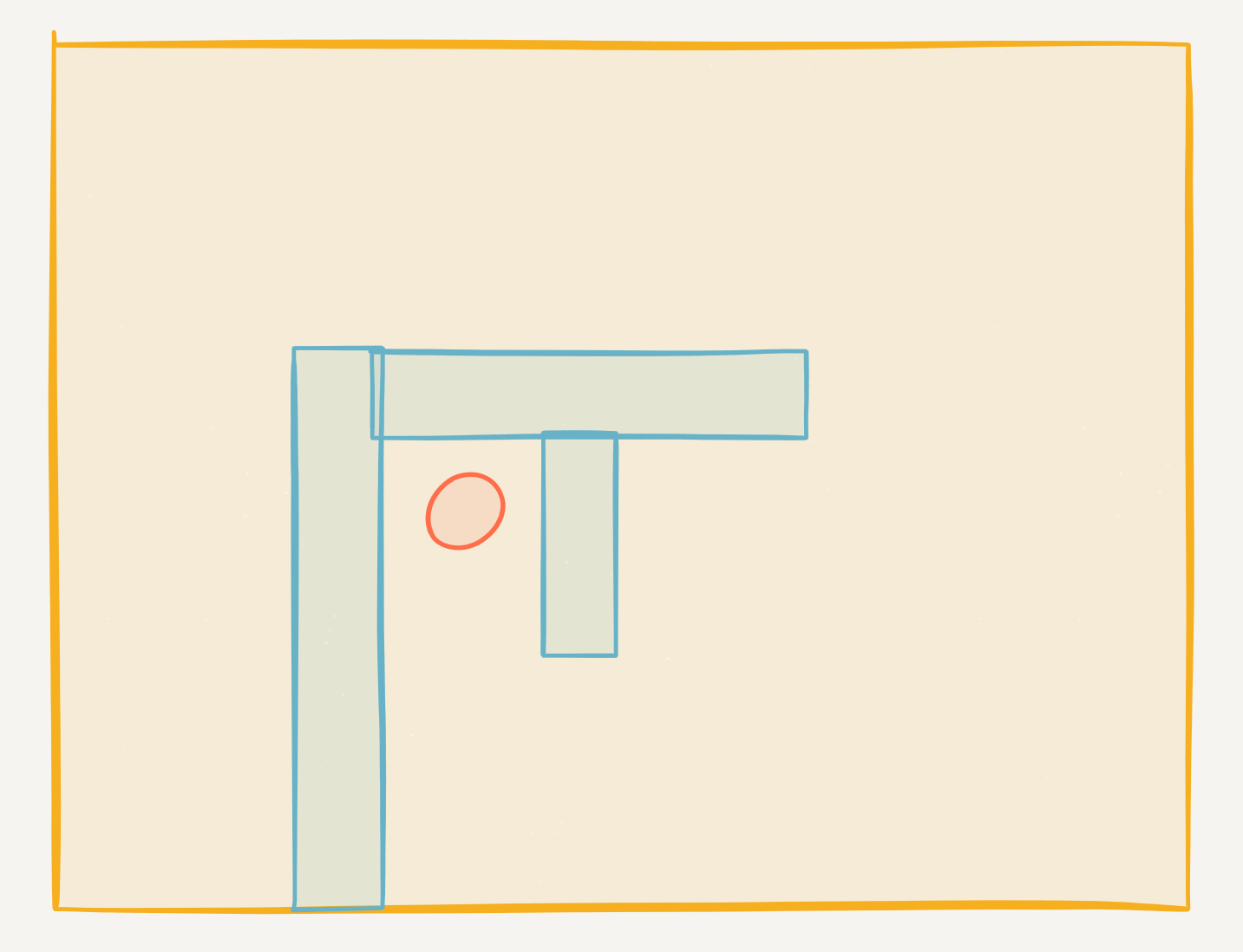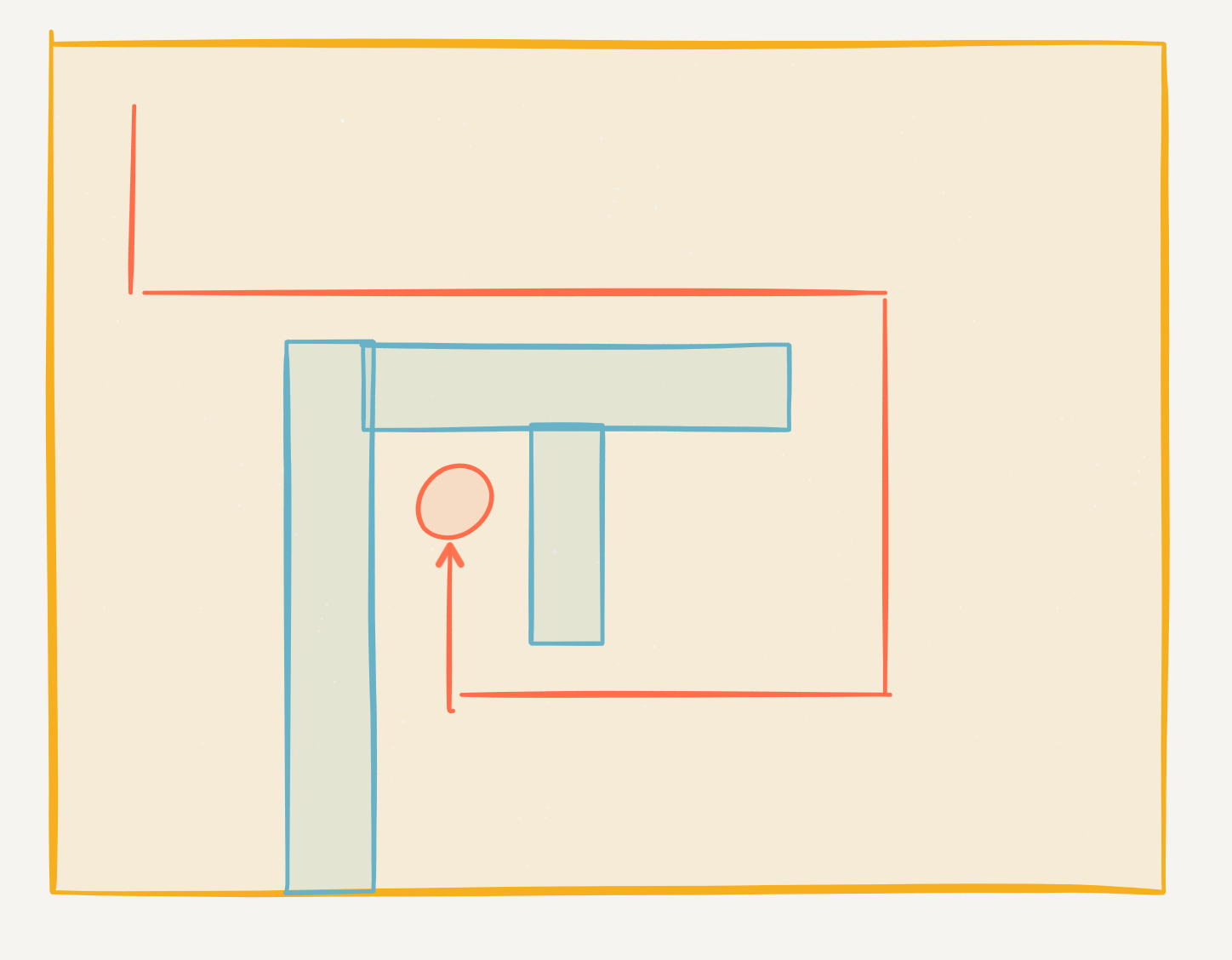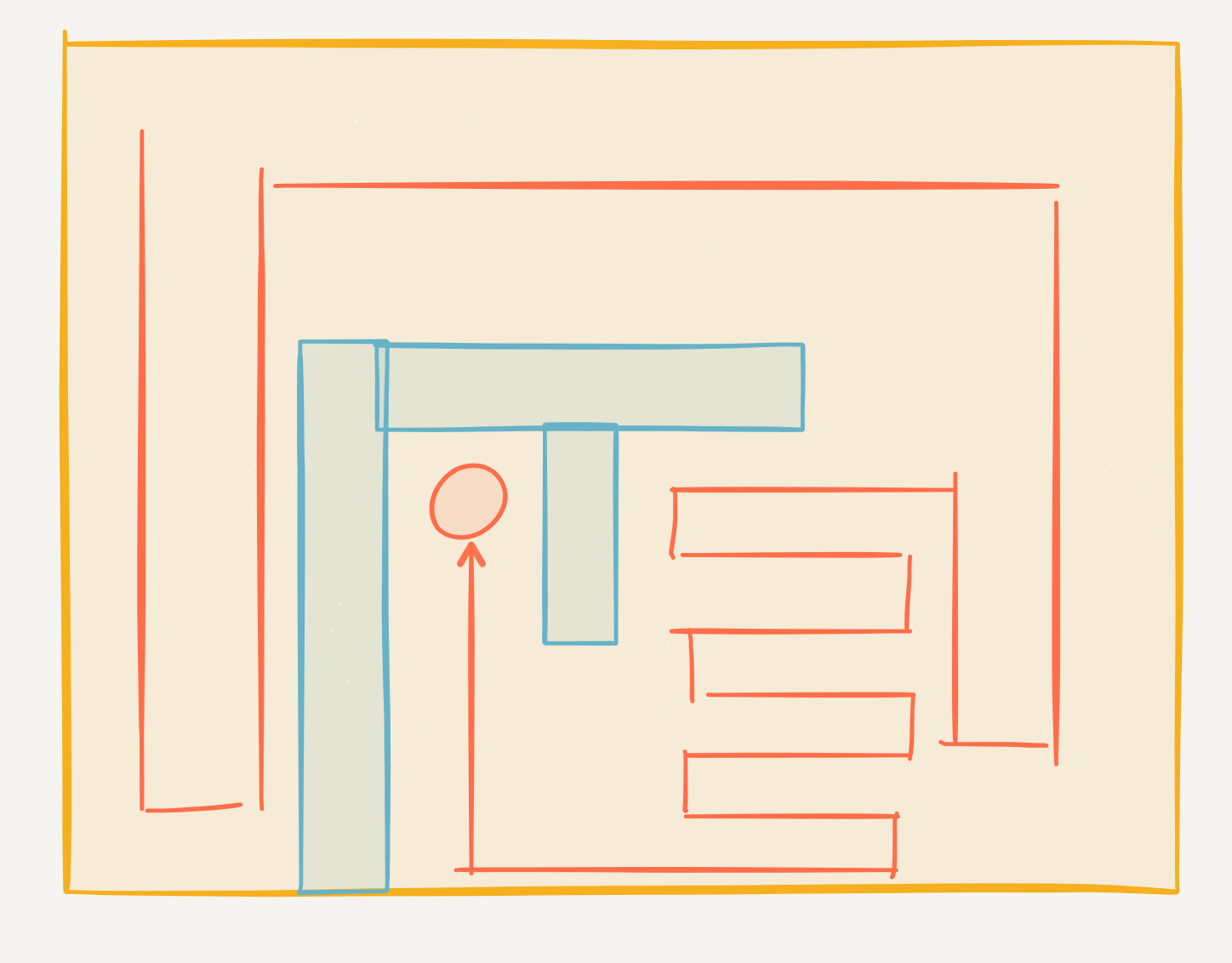• 定义

DFS）,是一种搜索算法，经常用在，树（Tree）和图（Graph）的搜索中，不过不知道树和图的也没关系，这篇文章不会显性的出现这些东西。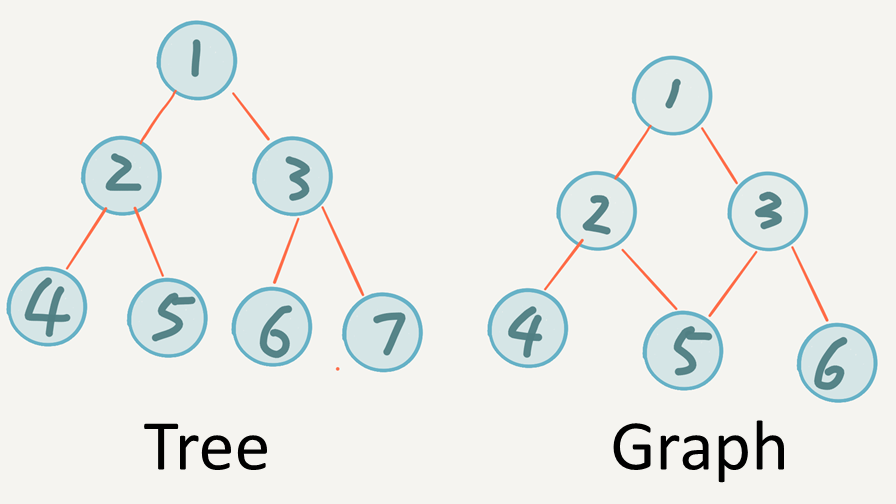dfs(currentStep)， 那么下一步不就是 dfs(currentStep+1)喽~ 看到这里有一定基础的人已经猜到了，这个算法要用到递归，没错。

``void dfs(int currentStep){``  if 到达边界``      return``  for each move //尝试每一种可能走法``  {``      dfs(currentStep+1); //进行下一步处理``  }``  return``}``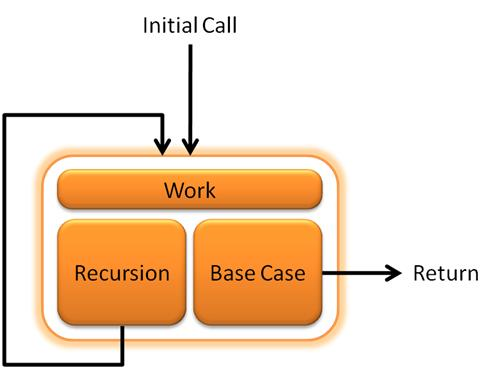1.基准情形（Base Case） ，必须总要有某些基准的清醒，在这个情形中，不执行递归就能求解。
2.不断推进（Making Progress）
，对于需要递归求解的情形，递归调用必须总能够朝着一个基准情形推进。这样的话，不断推进到基准情形，到达基准情形的时候递归才会推出，得到返回值。

• 实现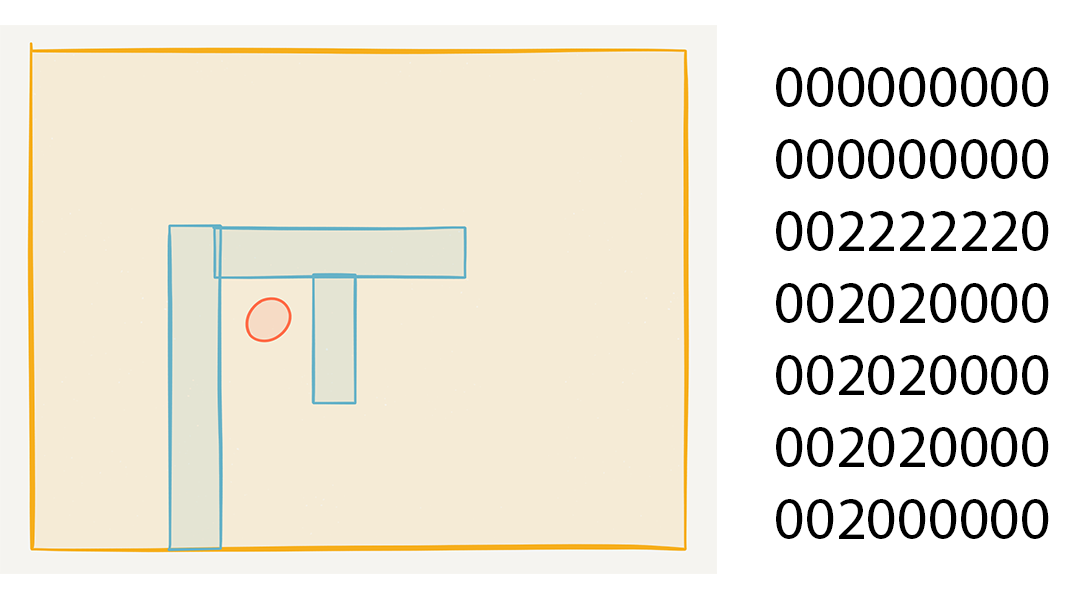``public class MapPoint {``    public static int MAP_PONIT_OCCUPIED = 1;``    public static int MAP_PONIT_EMPTY = 0;``    public static int MAP_PONIT_OBSTRUCTION = 2;``    private boolean occupied = false;``    private int type;``    public MapPoint(int type){} ``    public int getType() {}``    public void setType(int type) {}``    public boolean isOccupied() {}``}``

``public class Map {``    private MapPoint[][] mapArr;``    int mapHeight;``    int mapWidth;``    public Map (String path){}``    public MapPoint[][] getMapArr() {} //得到地图二维数组``    public void setPoint(int i,int j,int type){} //设置点类型``    public MapPoint getPoint(int i,int j){} //获取指定坐标的点``    public void printMap(){} //打印地图``    public void readMapFromFile(String path){} //从文件读取地图``}``

Map类实现了对地图的各种操作：读取，打印输出，地图点编辑都由这个类处理

• dfs函数

``void dfs(int x, int y, int step){}``

``void dfs(int x, int y, int step){``      if (x == targetX && y == targetY){ //到达目标位置``            if (depth<minDepth){ //更新最小步数``                minDepth = depth;``            }``            return; //这个return用来结束当前递归，一定别忘了！！！``     }``}``

``int[][] next = {``        {0,1},//Right``        {1,0},//Down``        {0,-1},//Left``        {-1,0}//Up``};``

``for (int k=0;k<=3;k++){``      //生成下一点的坐标``    nextX = x + next[k];``    nextY = y + next[k];``      //判断是否出现越界窗框``    if (nextX<0 || nextX>map.mapHeight-1 || nextY < 0 || nextY>map.mapWidth-1){``        continue; //结束当前进程而不退出循环``    }``      //判断这个点有没有被占用，或是障碍物（这个逻辑已在MapPoint类中封装好了）``    if (!map.getPoint(nextX,nextY).isOccupied()){``        map.setPoint(nextX,nextY,1); //标记这个点已经被走过了 ``        dfs(nextX,nextY,step+1); //调用自身，尝试下一个点``        map.setPoint(nextX,nextY,0); //尝试结束后取消这个点的占用标记``    }``}``

List）来实现（如果用栈（Stack）的话输出的坐标就是从最后到第一个，效果不好，要是用队列的话，错的更是没边了。。队列不能移除最后一个元素，只能移除第一个），我这样做只是为了速度，其实用个一位数组也行啊，只不过这样做太傻了，编程的时候能正确的使用数据结构是很重要的：

• 如果到达终点就把当前的链表复制一份当做最优路线
• 每次标记点已经走过的时候，再把这个点插入到双向链表里
• 尝试结束后取消点的占用标记的时候顺便移除双向链表的最后插入的元素

`` public static void main(String[] args) {``     Map map = new Map("C:\\Users\\Mike\\Desktop\\map.txt");``     RouteFinder finder = new RouteFinder(map,0,0,4,3);``     finder.findBestRoute();`` }``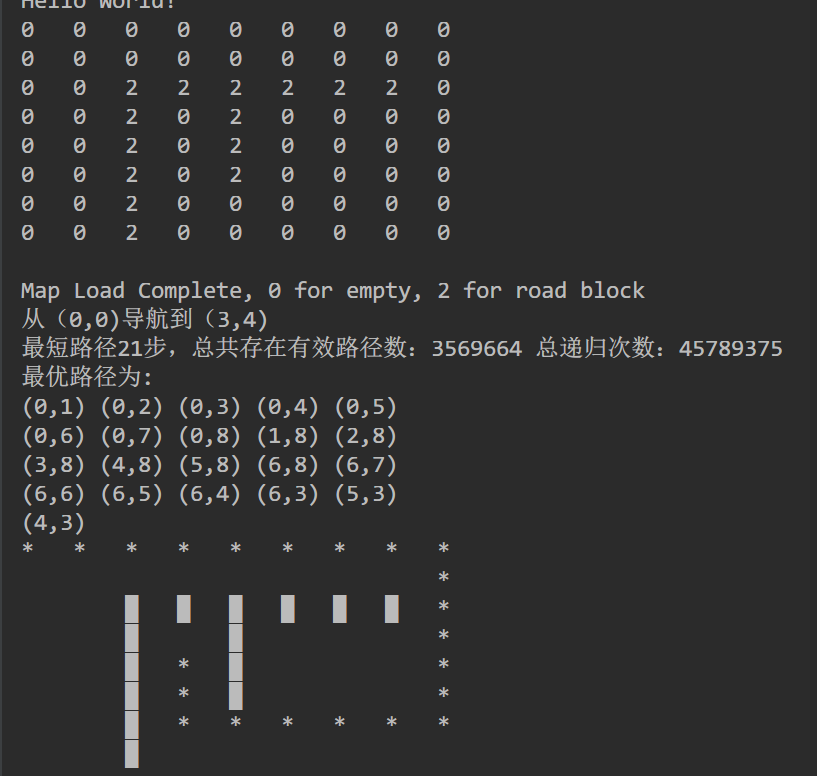，编程的初学者都能做到，3个嵌套for循环。。。 求出 1， 2， 3，4 ，5 五个数的全排列，好，简单，5个嵌套for循环。。。 行，